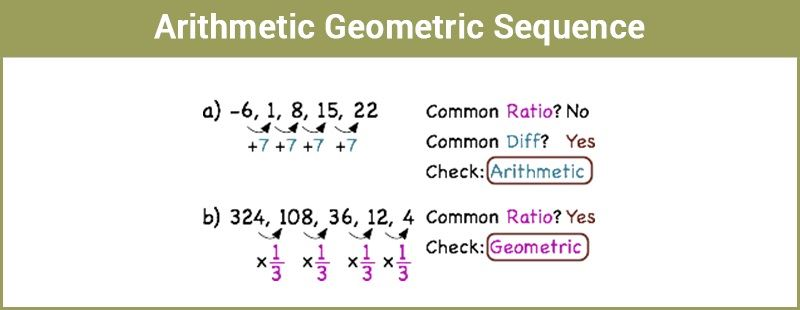# Arithmetic Geometric SequenceArithmetic Geometric sequence is the fusion of an arithmetic sequence and a geometric sequence. In this article, we are going to discuss the arithmetic-geometric sequences and the relationship between them. Also, get the brief notes on the geometric mean and arithmetic mean with more examples.

## What is Arithmetic Sequence?

An arithmetic sequence is of the form:

$$\begin{array}{l}a, ~a+d,~ a+2d,~……~a~+~(n~-~2)d,~ a~+~(n~-~1)d\end{array}$$
;

where

$$\begin{array}{l}a\end{array}$$
is the first term,

$$\begin{array}{l}d\end{array}$$
is the common difference

$$\begin{array}{l}a~+~(n~-~1)d\end{array}$$
is
$$\begin{array}{l}n^{th}\end{array}$$
term of A.P.

## What is a Geometric Sequence?

Similarly, a geometric sequence is of the form
$$\begin{array}{l}b,~ br, ~br^2~,~ ……~br^{n~-~2}, br^{n~-~1}\end{array}$$

here

$$\begin{array}{l}b\end{array}$$
is the first term,

$$\begin{array}{l}r\end{array}$$
is the common ratio

$$\begin{array}{l}br^{n~-~1}\end{array}$$
is
$$\begin{array}{l}n^{th}\end{array}$$
term of the G.P.

## What is Arithmetic Geometric Sequence?

Let
$$\begin{array}{l}a_1,~ a_2, ~a_3,~ …….~a_n\end{array}$$
be an A.P and
$$\begin{array}{l}b_1,~ b_2,~ b_3,~ …….~b_n\end{array}$$
be a G.P
Then,
$$\begin{array}{l}a_1 ~b_1,~ a_2~ b_2,~ a_3~ b_3,~ ……, ~a_n~ b_n\end{array}$$
is called as an arithmetic geometric sequence and it is of the form,
$$\begin{array}{l}ab,~ (a~+~d)br, ~(a~+~2d)br^2,~ …….,~[a~+~(n-2)d]~br^{n~-~2}, ~[a~+~(n~-~1)d]br^{n~-~1}\end{array}$$

Sum of n terms of the above sequence is found as follows:

$$\begin{array}{l}S_n =ab+ (a+d)br +(a+2d)br^2+…….+ [a+(n-2)d]br^{n-2}+ [a+(n-1)d]br^{n-1}\end{array}$$
—- (1)

Now, multiplying each term with

$$\begin{array}{l}r\end{array}$$
gives,

$$\begin{array}{l}rS_n = abr+(a+d)br^2+ (a+2d)br^3+….+[a+(n-2)d]br^{n-1}+[a+(n-1)d]br^n\end{array}$$
––(2)

Subtract equation (2) from equation (1), we get

$$\begin{array}{l}S_n~-~rS_n = ab~+~dbr~+~dbr^2~+~dbr^3~+~ ……~+ ~dbr^{n-1}~–~[a~+~(n~-~1)d]br^n\end{array}$$
—(3)

In the above series, if we exclude the first term and the last term,

$$\begin{array}{l}dbr~+~dbr^2~+~ dbr^3~ +~ ……….~+ ~dbr^{n~-~1}\end{array}$$
is a G.P
Sum of
$$\begin{array}{l}n\end{array}$$
terms of G.P is a
$$\begin{array}{l}\frac{1~-~r^n}{1~-~r}\end{array}$$
,
Therefore,
$$\begin{array}{l}dbr~+~dbr^2~+~ dbr^3~ +~ ……….~+ ~dbr^{n~-~1}\end{array}$$
=
$$\begin{array}{l}dbr\frac{1~-~r^{n~-~1}}{1~-~r}\end{array}$$

Now, (3) becomes as,

(1-r) Sn =
$$\begin{array}{l}ab ~+~ dbr\frac{1~-~r^{n~-~1}}{1~-~r}~-~[a~+~(n~-~1)d]br^n\end{array}$$
,

where

$$\begin{array}{l}r~≠~0\end{array}$$

Sn =
$$\begin{array}{l}\frac{ab}{1~-~r}~+~dbr\frac{1~-~r^{n~-~1}}{(1-r)^2}~ -~\frac{[a~+~(n-1)d]br^n}{1~-~r}\end{array}$$

If the common ratio of the sequence lies between -1 and 1, then

$$\begin{array}{l} \lim\limits_{n→∞}~r^n\end{array}$$
=
$$\begin{array}{l}0\end{array}$$

Therefore, the sum of infinite terms of the sequence in (1) is,

S =
$$\begin{array}{l}\frac{ab}{1~-~r} ~+ ~\frac{dbr}{(1-r)^2}\end{array}$$
[-1<r<1]

## Geometric Mean

Consider two positive numbers a and b, the geometric mean of these two numbers is

$$\begin{array}{l}√ab\end{array}$$
.

For example; Geometric mean of 3 and 27 is √(3×27)=9

The numbers 3, 9, 27 is in a G.P with common ratio 3.

In general; between 2 positive numbers a and b, we can insert as many numbers as we like such that the resulting sequence forms a G.P.

Let G1, G2, G3……Gn be the n numbers inserted between the positive numbers a and b such that,

a,G1,G2,G3……Gn forms a G.P. “a” is the first term and b is (n+2)th term.

b = ar{n+1},

$$\begin{array}{l} r = \left(\frac{b}{a}\right)^{\frac{1}{n~+~1}}\end{array}$$

It gives, G1 = ar =
$$\begin{array}{l}a\left(\frac{b}{a}\right)^{\frac{1}{n~+~1}}\end{array}$$
G2 = ar2 =
$$\begin{array}{l}a\left(\frac{b}{a}\right)^{\frac{2}{n~+~1}}\end{array}$$
G2 = ar3 =
$$\begin{array}{l}a(\frac{b}{a})^{\frac{3}{n~+~1}}\end{array}$$
Gn = arn =
$$\begin{array}{l}a(\frac{b}{a})^{\frac{n}{n~+~1}}\end{array}$$

### Relation Between Arithmetic Mean (A.M) and Geometric Mean (G.M)

Consider two positive numbers
$$\begin{array}{l}a\end{array}$$
and
$$\begin{array}{l}b\end{array}$$
;
$$\begin{array}{l}A.M\end{array}$$
=
$$\begin{array}{l}\frac{a~+~b}{2}\end{array}$$
$$\begin{array}{l}G.M\end{array}$$
=
$$\begin{array}{l}√ab\end{array}$$
$$\begin{array}{l}A.M~-~G.M\end{array}$$
=
$$\begin{array}{l}\frac{a~+~b}{2}~-~√ab\end{array}$$
=
$$\begin{array}{l}\frac{a~+~b~-~2√ab}{2}\end{array}$$
$$\begin{array}{l}A.M~-~G.M\end{array}$$
=
$$\begin{array}{l}\frac{(√a~-~√b)^2}{2}\end{array}$$
which is greater than or equal to 0.

Therefore,

$$\begin{array}{l}A.M~≥~G.M\end{array}$$

### Arithmetic Geometric Sequence Example

Example 1:

Insert 3 numbers between 4 and 64 so that the resulting sequence forms a G.P.

Solution:

Let G1,G2,G3 be the three numbers to be inserted between 4 and 64,

$$\begin{array}{l} r = (\frac{b}{a})^{\frac{1}{n~+~1}} = (\frac{64}{4})^{\frac{1}{3~+~1}} = (16)^{\frac{1}{4}} = 2\end{array}$$

G1 =

$$\begin{array}{l}4~×~2\end{array}$$
= 8

G2 =

$$\begin{array}{l}8~×~2\end{array}$$
= 16

G3 =

$$\begin{array}{l}16~×~2\end{array}$$
= 32

Example 2:

A.M and G.M of two numbers are 5 and 4 respectively. Find the two numbers.

Solution:

Let the numbers be
$$\begin{array}{l}a\end{array}$$
and
$$\begin{array}{l}b\end{array}$$
,
$$\begin{array}{l}\frac{a~+~b}{2}\end{array}$$
=
$$\begin{array}{l}5\end{array}$$
,
$$\begin{array}{l}a~+~b\end{array}$$
=
$$\begin{array}{l}5~×~2\end{array}$$
=
$$\begin{array}{l}10\end{array}$$
—(1)
$$\begin{array}{l}√ab\end{array}$$
=
$$\begin{array}{l}4\end{array}$$
,
$$\begin{array}{l}ab\end{array}$$
=
$$\begin{array}{l}16\end{array}$$
$$\begin{array}{l}(a~+~b)^2~-~(a~-~b)^2\end{array}$$
=
$$\begin{array}{l}4ab\end{array}$$
$$\begin{array}{l}{10}^2~-~(a~-~b)^2\end{array}$$
=
$$\begin{array}{l}4~×~16\end{array}$$
=
$$\begin{array}{l}64\end{array}$$
$$\begin{array}{l}(a~-~b)^2\end{array}$$
=
$$\begin{array}{l}100~-~64\end{array}$$
=
$$\begin{array}{l}36\end{array}$$
$$\begin{array}{l}a~-~b\end{array}$$
=
$$\begin{array}{l}±6\end{array}$$
—(2)

By solving (1) and (2),

$$\begin{array}{l}2a\end{array}$$
=
$$\begin{array}{l}16\end{array}$$
,
$$\begin{array}{l}a\end{array}$$
=
$$\begin{array}{l}\frac{16}{2}\end{array}$$
=
$$\begin{array}{l}8\end{array}$$
$$\begin{array}{l}b\end{array}$$
=
$$\begin{array}{l}10~-~a\end{array}$$
=
$$\begin{array}{l}10~-~8\end{array}$$
=
$$\begin{array}{l}2\end{array}$$

Thus, some examples of the arithmetic geometric progression are discussed above. To learn different kinds of progressions in Maths, download BYJU’S – The Learning App.